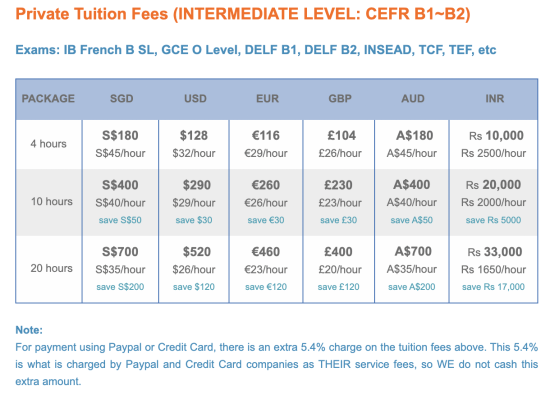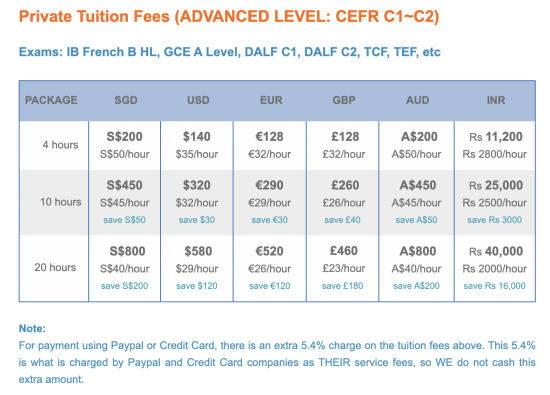# Fees

## French Tuition Fees

• There is a one-time registration fee of SGD30/USD23/€20/£17/AUD30.
• All learning materials from the teacher are provided FREE of charge.
• The minimum hours required for us to set up a program is 4 hours.
• For purchases above 20 hours, 20-hour rate applies. For purchases below 4 hours, 4-hour rate applies.
• Do read our Terms and Conditions of Service below; it covers Class booking, Lateness, Absence, Replacement classes, Homework, Refund policy, Use-by date, etc.
• The prices listed below are for Private Classes (1:1).
• Fees for Semi-Private Classes (2 students in a class):
– 2 (two) students in a class= (listed price + 60% of listed price) / 2
Example: 2 Primary students taking a 10-hour Package:
Per student = [SGD350 + (60% x SGD350)] / 2 = (SGD350 + SGD210) / 2 = SGD560 / 2 = SGD280
• Fees for Group Classes (3 or more students in a class):
– 3 or more students in a class= (listed price + 50% of listed price) / 3
Example: 3 Primary students taking a 10-hour Package:
Per student = [SGD350 + (50% x SGD350)] / 3 = (SGD350 + SGD175) / 3 = SGD525 / 3 = SGD175### Terms & Conditions

• There is a one-time registration fee of SGD30/USD23/€20/£17/AUD30.
• All learning materials from the teacher are provided FREE of charge.
• The minimum hours required for us to set up a program is 4 hours.
• For purchases above 20 hours, 20-hour rate applies. For purchases below 4 hours, 4-hour rate applies.
• Do read our Terms and Conditions of Service below; it covers Class booking, Lateness, Absence, Replacement classes, Homework, Refund policy, Use-by date, etc.
• The prices listed below are for Private Classes (1:1).
• Fees for Semi-Private Classes (2 students in a class):
– 2 (two) students in a class= (listed price + 60% of listed price) / 2
Example: 2 Primary students taking a 10-hour Package:
Per student = [SGD350 + (60% x SGD350)] / 2 = (SGD350 + SGD210) / 2 = SGD560 / 2 = SGD280
• Fees for Group Classes (3 or more students in a class):
– 3 or more students in a class= (listed price + 50% of listed price) / 3
Example: 3 Primary students taking a 10-hour Package:
Per student = [SGD350 + (50% x SGD350)] / 3 = (SGD350 + SGD175) / 3 = SGD525 / 3 = SGD175
Scroll to Top